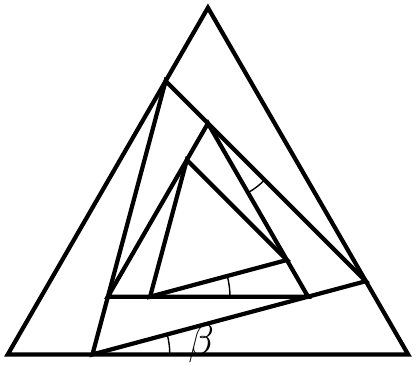###### back to index | new

An infinite number of equilateral triangles are constructed as shown on the right. Each inner triangle is inscribed in its immediate outsider and is shifted by a constant angle $\beta$. If the area of the biggest triangle equals to the sum of areas of all the other triangles, find the value of $\beta$ in terms of degrees.For $-1 < r < 1$, let $S(r)$ denote the sum of the geometric series $$12+12r+12r^2+12r^3+\cdots .$$Let $a$ between $-1$ and $1$ satisfy $S(a)S(-a)=2016$. Find $S(a)+S(-a)$.

A strictly increasing sequence of positive integers $a_1$, $a_2$, $a_3$, $\cdots$ has the property that for every positive integer $k$, the subsequence $a_{2k-1}$, $a_{2k}$, $a_{2k+1}$ is geometric and the subsequence $a_{2k}$, $a_{2k+1}$, $a_{2k+2}$ is arithmetic. Suppose that $a_{13} = 2016$. Find $a_1$.

Initially Alex, Betty, and Charlie had a total of $444$ peanuts. Charlie had the most peanuts, and Alex had the least. The three numbers of peanuts that each person had formed a geometric progression. Alex eats $5$ of his peanuts, Betty eats $9$ of her peanuts, and Charlie eats $25$ of his peanuts. Now the three numbers of peanuts each person has forms an arithmetic progression. Find the number of peanuts Alex had initially.

Triangle $ABC_0$ has a right angle at $C_0$. Its side lengths are pariwise relatively prime positive integers, and its perimeter is $p$. Let $C_1$ be the foot of the altitude to $\overline{AB}$, and for $n \geq 2$, let $C_n$ be the foot of the altitude to $\overline{C_{n-2}B}$ in $\triangle C_{n-2}C_{n-1}B$. The sum $\sum_{i=1}^\infty C_{n-2}C_{n-1} = 6p$. Find $p$.

The sequences of positive integers $1,a_2, a_3,...$ and $1,b_2, b_3,...$ are an increasing arithmetic sequence and an increasing geometric sequence, respectively. Let $c_n=a_n+b_n$. There is an integer $k$ such that $c_{k-1}=100$ and $c_{k+1}=1000$. Find $c_k$.

For $1 \leq i \leq 215$ let $a_i = \dfrac{1}{2^{i}}$ and $a_{216} = \dfrac{1}{2^{215}}$. Let $x_1, x_2, ..., x_{215}$ be positive real numbers such that $\sum_{i=1}^{215} x_i=1$ and $\sum_{i \leq i < j \leq 216} x_ix_j = \dfrac{107}{215} + \sum_{i=1}^{216} \dfrac{a_i x_i^{2}}{2(1-a_i)}$. The maximum possible value of $x_2=\dfrac{m}{n}$, where $m$ and $n$ are relatively prime positive integers. Find $m+n$.

Starting at the origin, a bug crawls 1 unit up, 2 units right, 3 units down and 4 units left. From this new point, the bug repeats this entire sequence of four moves 2015 more times, for a total of 2016 times. The coordinates of the bug’s final location are $(a, b)$. What is the value of $a + b$?

For each positive integer $n$, $a_n = 9n + 2$ and $b_n = 7n + 3$. If the values common to both sequences are written as a sequence, the $n^{th}$ term of that sequence can be expressed as $pn + q$. What is the value of $p − q$?

Let the sum of first $n$ terms of arithmetic sequence $\{a_n\}$ be $S_n$, and the sum of first $n$ terms of arithmetic sequence $\{b_n\}$ be $T_n$. If $\frac{S_n}{T_n}=\frac{2n}{3n+7}$, compute the value of $\frac{a_8}{b_6}$.

Suppose every term in the sequence $$1, 2, 1, 2, 2, 2, 1, 2, 2, 2, 2, 2, 1, \cdots$$ is either $1$ or $2$. If there are exactly $(2k-1)$ twos between the $k^{th}$ one and the $(k+1)^{th}$ one, find the sum of its first $2014$ terms.

Let $c_1, c_2, c_3, \cdots$ be a series of concentric circles whose radii form a geometric sequence with common ratio as $r$. Suppose the areas of rings which are formed by two adjacent circles are $S_1, S_2, S_3, \cdots$. Which statement below is correct regarding the sequence $\{S_n\}$? A) It is not a geometric sequence B) It is a geometric sequence and its common ratio is $r$ C) It is a geometric sequence and its common ratio is $r^2$ D) It is a geometric sequence and its common ratio is $r^2-1$

Given the sequence $\{a_n\}$ satisfies $a_n+a_m=a_{n+m}$ for any positive integers $n$ and $m$. Suppose $a_1=\frac{1}{2013}$. Find the sum of its first $2013$ terms.

Let sequence $\{a_n\}$ satisfy $a_1=2$ and $a_{n+1}=\frac{2(n+2)}{n+1}a_n$ where $n\in \mathbb{Z}^+$. Compute the value of $$\frac{a_{2014}}{a_1+a_2+\cdots+a_{2013}}$$

Let $a_1, a_2,\cdots, a_n > 0, n\ge 2,$ and $a_1+a_2+\cdots+a_n=1$. Prove $$\frac{a_1}{2-a_1} + \frac{a_2}{2-a_2}+\cdots+\frac{a_n}{2-a_n}\ge\frac{n}{2n-1}$$

Suppose all the terms in a geometric sequence $\{a_n\}$ are positive. If $|a_2-a_3|=14$ and $|a_1a_2a_3|=343$, find $a_5$.

Suppose no term in an arithmetic sequence $\{a_n\}$ equals $0$. Let $S_n$ be the sum of its first $n$ terms. If $S_{2n-1} = a_n^2$, find the expression for its $n^{th}$ term $a_n$.

Let $S_n$ be the sum of first $n$ terms in sequence $\{a_n\}$ where $$a_n=\sqrt{1+\frac{1}{n^2}+\frac{1}{(n+1)^2}}$$ Find $\lfloor{S_n}\rfloor$ where the floor function $\lfloor{x}\rfloor$ returns the largest integer not exceeding $x$.

Let $\alpha$ and $\beta$ be the two roots of the equation $x^2 -x - 1=0$. If $$a_n = \frac{\alpha^n - \beta^n}{\alpha -\beta}\quad(n=1, 2, \cdots)$$ Show that - For any positive integer $n$, it always hold $a_{n+2}=a_{n+1}+a_n$ - Find all positive integers $a, b$ $( a < b )$ satisfying $b\mid a_n-2na^n$ holds for any positive integer $n$

Let $\{a_n\}$ be an increasing geometric sequence satisfying $a_1+a_2=6$ and $a_3+a_4=24$. Let $\{b_n\}$ be another sequence satisfying $b_n=\frac{a_n}{(a_n-1)^2}$. If $T_n$ is the sum of first $n$ terms in $\{b_n\}$, show that for any positive integer $n$, it always holds that $T_n < 3$.

Given a sequence $\{a_n\}$, if $a_n\ne 0$, $a_1=1$, and $3a_na_{n-1}+a_n+a_{n-1}=0$ for any $n\ge 2$, find the general term of $a_n$.

If a sequence $\{a_n\}$ satisfies $a_1=1$ and $a_{n+1}=\frac{1}{16}\big(1+4a_n+\sqrt{1+24a_n}\big)$, find the general term of $a_n$.

Let sequence $\{a_n\}$ satisfy $a_0=1$ and $a_n=\frac{\sqrt{1+a_{n-1}^2}-1}{a_{n-1}}$. Prove $a_n > \frac{\pi}{2^{n+2}}$.

Show that $1+3+6+\cdots+\frac{n(n+1)}{2}=\frac{n(n+1)(n+2)}{6}$.

Show that $1+4+7+\cdots+(3n-2)=\frac{n(3n-1)}{2}$